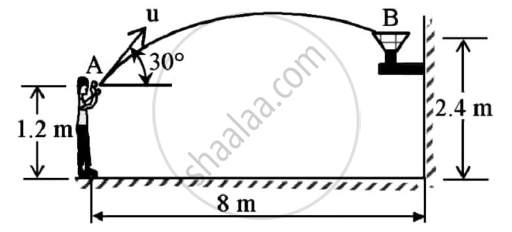Advertisement Remove all ads

# Determine the Speed at Which the Basket Ball at a Must Be Thrown at an Angle 30o So that If Makes It to the Basket at B. Also Find at What Speed It Passes Through the Hoop. - Engineering Mechanics

Answer in Brief

Determine the speed at which the basket ball at A must be thrown at an angle 30o so that if makes it to the basket at B.
Also find at what speed it passes through the hoop.Advertisement Remove all ads

#### Solution

Given : θ=30o
To find : Speed at which basket ball must be thrown
Solution :
Assume that the basket ball be thrown with initial velocity u and it takes time t to reach B
HORIZONTAL MOTION
Here the velocity is constant
8 = ucos30 x t
t=8/(ucos30)=9.2376/u……..(1)
vB = ucos30 (Since velocity is constant in horizontal motion) ………(2)
VERTICAL MOTION
Initial vertical velocity (uv) = usin30 =0.5u …….(3)
Vertical displacement(s) = 2.4 - 1.2 = 1.2
t=9.2376/u
Using kinematical equation :
s = ut + 1/2 x at2
1.2=u/2x9.2376/u-1/2x9.81x((9.2376)/u)^2
u2=122.4289
u=11.0648 m/s
uv=0.5u (From 3)
uv = 0.5 x 11.0648
= 5.5324 m/s
Using kinematical equation
vv2 = uv2 + 2as
vv2 = 5.53242 - 2 x 9.81 x 1.2
vv = 2.6622 m/s
vh = 11.0648cos30 = 9.5824 m/s (From 2)
v_B=sqrtV_v^2+v_h^2
vB = 9.9441 m/s

apha=tan^-1((2.6577)/(9.5824))
= 15.5015°
Speed at which the basket-ball at A must be thrown = 11.0648 m/s (30° in first quadrant)
Speed at which the basket-ball passes through the hoop = 9.9441 m/s(15.5015° in fourth quadrant)

Concept: Motion Curves (a-t, v-t, s-t curves)
Is there an error in this question or solution?
Advertisement Remove all ads

#### APPEARS IN

Advertisement Remove all ads
Advertisement Remove all ads
Share
Notifications

View all notifications

Forgot password?